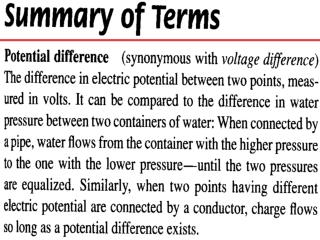DownloadDownload PresentationHydraulic circuit

# Hydraulic circuit

Télécharger la présentation## Hydraulic circuit

- - - - - - - - - - - - - - - - - - - - - - - - - - - E N D - - - - - - - - - - - - - - - - - - - - - - - - - - -
##### Presentation Transcript

1. Hydraulic circuit Electric circuit

2. Direct & Alternating Current Diode  AC  DC Diode  AC  DC

3. Electric Current

4. Series Circuit Req = R1 + R2 + R3

5. Parallel Circuit 1/Req = 1/R1 + 1/R2 + 1/R3

6. -- Questions -- MULTIPLE CHOICE. Choose the one alternative that best completes the statement or answers the question.

7. 1) The source of electrons lighting an incandescent ac light bulb is • A) the wire leading to the lamp. • B) electrical outlet. • C) the source voltage. • D) the power company. • E) atoms in the light bulb filament. • 2) A circuit is powered with a battery. Charge flows • A) from the negative battery terminal to the positive terminal. • B) through both the battery and the rest of the circuit. • C) after a couple seconds passes. • D) out of the battery and into the circuit. • E) none of these.

8. 3) If you plug an electric toaster rated at 110 V into a 220-V outlet, the current in the toaster will be about • A) twice what it should be. • B) the same as if it were plugged into 110 V. • C) more than twice what it should be. • D) half what it should be. • 4) Electrons move in an electrical circuit • A) by being bumped by other electrons. • B) because the wires are so thin. • C) by colliding with molecules. • D) by interacting with an established electric field. • E) none of these.

9. 5) Two lamps, one with a thick filament and one with a thin filament, are connected in parallel to a battery. The voltage is greatest across the lamp with the • A) thick filament. • B) thin filament. • C) both voltages are the same. • 6) A capacitor is used to store • charge. • energy. • both. • D) neither.

10. 7) What is the power rating of a light bulb if 0.8 A flow through it when it is connected to a 120-volt outlet? • 12 W • 15 W • 60 W • 96 W • E) 120 W • 8) A woman experiences an electrical shock. The electrons making the shock come from the • A) hairdryer. • B) power plant. • C) woman's body. • D) electric field in the air. • E) ground.

11. 9) A 10-ohm resistor has a 5-A current in it. What is the voltage across the resistor? • A) 5 V • B) 10 V • C) 15 V • D) 20 V • E) more than 20 V • 10) Connect a pair of lamps in series and you draw current from the connected battery. Connect the same lamps in parallel and you draw • more current. • B) the same current. • C) less current. • D) ...sometimes more, sometimes less.

12. 11) Two lamps, one with a thick filament and one with a thin filament, are connected in series. The current is greater in the lamp with the • thick filament. • thin filament. • C) the same in each lamp. • 12) The current through a 10-ohm resistor connected to a 120-V power supply is • A) 1 A. • B) 10 A. • C) 12 A. • D) 120 A. • E) none of these.

13. 13) When a pair of 1-ohm resistors are connected in series, their combined resistance is • A) 2 ohms, and when in parallel, 1/2 ohm. • B) 1 ohm, and when connected in parallel, 2 ohms. • C) 2 ohms, and when in parallel, 1 ohm. • D) 1/2 ohm, and when in parallel, 2 ohms. • E) none of these. • 14) Which statement is correct? • A) Resistance is established across a circuit. • B) Current causes voltage. • C) Voltage flows through a circuit. • D) Charge flows in a circuit.

14. 15) An electrical diode is useful for • A) voltage modification. • B) changing ac to dc. • C) limiting current. • D) storing electrical energy. • E) boosting voltage.

15. -- Answers -- MULTIPLE CHOICE. The one alternative that best completes the statement or answers the question.